Maths-
General
Easy

Question

# The sum of the subtangent and subnormal to the curve  at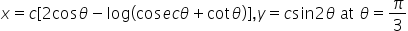is

##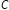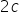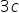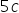Hint:

## The correct answer is:### x = c[2cosθ - log(cosecθ + cotθ)y = csin2θA tangent to the curve is given by the slope at the point. The slope is given by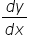let's differentiate x w.r.t θ.We will differentiate y w.r.t θNow, let us denote slope by mm = √3y1 =(y)θ=π/3 =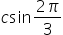So, the answer is 2c

For such questions, we should know the formula of subtangent and subnormal#### With Turito Foundation.#### Get an Expert Advice From Turito.